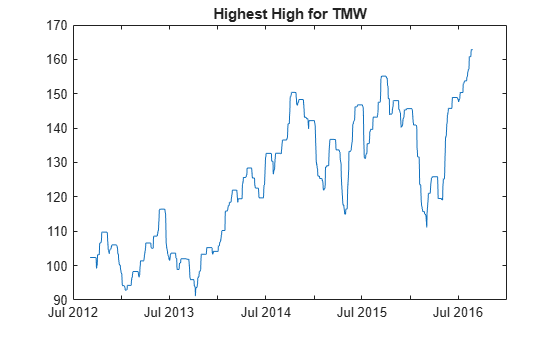# hhigh

Using a `fints` object for the `Data` argument of `hhigh` is not recommended. Use a matrix, `timetable`, or `table` instead for financial time series. For more information, see Convert Financial Time Series Objects fints to Timetables.

## Syntax

``values = hhigh(Data)``
``values = hhigh(___,Name,Value)``

## Description

example

````values = hhigh(Data)` generates a vector of highest high values from the series of high prices for the past n periods.```

example

````values = hhigh(___,Name,Value)` adds optional name-value pair arguments. ```

## Examples

collapse all

Load the file `SimulatedStock.mat`, which provides a timetable (`TMW`) for financial data for TMW stock.

```load SimulatedStock.mat values = hhigh(TMW); plot(values.Time,values.HighestHigh) title('Highest High for TMW')```## Input Arguments

collapse all

Data for high prices, specified as a matrix, table, or timetable. Timetables and tables with `M` rows must contain a variable named `'High'` (case insensitive).

Data Types: `double` | `table` | `timetable`

### Name-Value Arguments

Specify optional pairs of arguments as `Name1=Value1,...,NameN=ValueN`, where `Name` is the argument name and `Value` is the corresponding value. Name-value arguments must appear after other arguments, but the order of the pairs does not matter.

Before R2021a, use commas to separate each name and value, and enclose `Name` in quotes.

Example: `values = hhigh(TMW_HIGH,'NumPeriods',10)`

Moving window for the highest high calculation, specified as the comma-separated pair consisting of `'NumPeriods'` and a scalar positive integer.

Data Types: `double`

## Output Arguments

collapse all

Highest high series, returned with the same number of rows (`M`) and the same type (matrix, table, or timetable) as the input `Data`.

 Achelis, S. B. Technical Analysis from A to Z. Second Edition. McGraw-Hill, 1995.